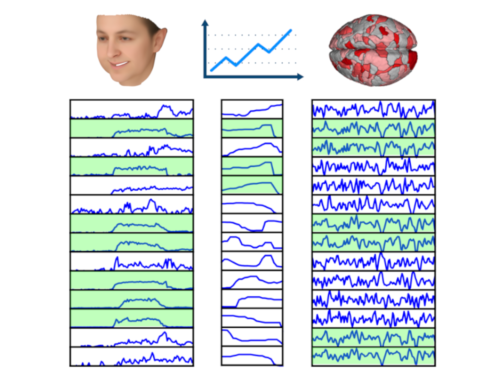•In CVPR’20 presented a new method (with code) for finding the largest subset of synchronized time series from a given set of time series. Specifically, we aim to find the largest subset of time series such that all pairs of in the subset are correlated at least by a (given) threshold value $\rho$. This is an NP-hard problem and the exact solution is, in general, unfeasible. We propose a new method, called SyncRef, for finding an approximate solution in an efficient…

• These three inequalities often tend to appear as a package in many textbooks about real analysis, signal processing or linear algebra. It is good to know the main reason that we see this package all the time, and to separate the role of each of these three fundamental inequalities. The overall reason is that, these inequalities are the key to generalize the facts about vectors in 2D/3D spaces and the Euclidean norm to higher dimensional spaces and (non-Euclidean) $p$-norms. Each of…